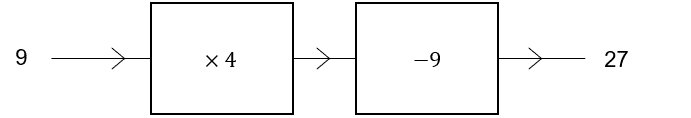# Function Machines

GCSELevel 4-5AQAEdexcelEdexcel iGCSEOCR

## Function Machines

A function machine takes an input, applies a series of mathematical operations to it, and then outputs a result. Function machines are quite a common question type, especially in foundation maths papers. There are 3 main types of questions you could be asked.

Make sure you are happy with the following topics before continuing.

Level 4-5GCSEAQAEdexcelOCREdexcel iGCSE

## Type 1: Input to Output

Here, you will be given an input and a function machine and then asked to produce the output.

Example: A function machine is shown below, what is the output if the input is $10$?Level 4-5GCSEAQAEdexcelOCREdexcel iGCSE

## Type 2: Output Back to Input

Here, you will be given an Output, a function machine, and then asked to find the Input which produced it.

Example: A function machine is shown below. What was the input if the output is $48$?If the output is $48$ we need to follow the operations of the function machine in reverse and do the opposite operation.

First we must reverse Operation 2 which is $\times 3$, this means we must $\div \, 3$.

$48 \div 3 = 16$

Next, Operation 1 which is $-10$, this means we must $+10$.

$16+10 = 26$

This shows the input was $26$

Level 4-5GCSEAQAEdexcelOCREdexcel iGCSE
Level 4-5GCSEAQAEdexcelOCREdexcel iGCSE

## Type 3: Creating Function Machines

You may be asked to create a function machine from an equation given.

Example: Draw a function machine below so that $b = \dfrac{a-3}{5}$

Here we have an input of $a$ and an output of $b$.

We must next look at the order of operations, for this we will use BIDMAS

As this is a fraction the first operation will be $a-3$

The Second would then be $(a-3) \div 5$

Using this we can create the function machine shown below.Level 4-5GCSEAQAEdexcelOCREdexcel iGCSE

## Example: Function Machines Problems

Inputting $5$ into the function machine below would give an output of $4$.

Find the value of $x$ in the function machine below.

[2 marks]So, if we input $5$ into the function machine then we must first add $\textcolor{orange}3$:

$5+3=8$

Now, the question tells us that the result of dividing $8$ by some number gives us $4$, so we divide by $\textcolor{orange}2$ to complete the function machine

$x = 2$

Level 4-5GCSEAQAEdexcelOCREdexcel iGCSE

## Function Machines Example Questions

a) Inputting $35$, we first multiply by $3$:

$35 \times 3 = 105$

Then, add $15$ to get

$105+15=120$

b) We must work backwards and do the opposite operations. So, first subtracting $15$ from the given output, we get

$48-15=33$

Then, dividing by $3$ we get,

$33\div 3=11$

Meaning that $11$ is the input required to give an output of $48$.Gold Standard Education

Inputting $-5$, we first multiply by $-2$:

$-5\times-2=10$

Then, adding $7$ we get,

$10+7=17$

Meaning that $-5$ is the input required to give an output of $17$.Gold Standard Education

Inputting $3x$, we first multiply by $\dfrac{1}{2}$

$3x\times\dfrac{1}{2}=\dfrac{3}{2}x$

Then, dividing by $3$ we get,

$\dfrac{3}{2}x\div3=\dfrac{1}{2}x$

Meaning that $3x$ is the input required to give an output of $\dfrac{1}{2}x$Gold Standard Education

Two operations are,

Multiplying by $4$, so $9 \times 4 = 36$

Subtracting $9$, so $36 - 9 = 27$Any combination of operations which produce an output of $27$ would be a correct answer.Gold Standard Education

We have to see what we get if we input $x$ into the function machine. First, multiplying $x$ by $12$,

$12\times x=12x$

Then, subtracting $25$ to get

$12x-25$

This is the output of inputting $x$ but we know the output is equal to $2x$, so we are left with the equation

$12x-25=2x$

Now we can solve this equation for $x$. Subtracting $2x$ from both sides, we get

$10x-25=0$

Adding $25$ to both sides, we get

$10x=25$

Finally, dividing both sides by $10$ we find $x$ to be

$x=25\div 10=2.5$Gold Standard Education

## Function Machines Worksheet and Example Questions

### (NEW) Function Machines Exam Style Questions - MME

Level 4-5GCSENewOfficial MMEProduct

£19.99

/month

Learn an entire GCSE course for maths, English and science on the most comprehensive online learning platform. With revision explainer videos & notes, practice questions, topic tests and full mock exams for each topic on every course, it’s easy to Learn and Revise with the MME Learning Portal.

Level 1-3GCSEKS3

Level 1-3GCSEKS3